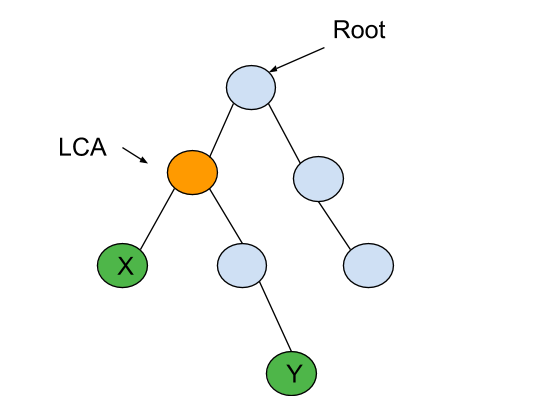New update is available. Click here to update.

# LCA Of Binary Tree

Last Updated: 6 Dec, 2020
Difficulty: Moderate

## PROBLEM STATEMENT

#### The LCA of ‘X’ and ‘Y’ in the binary tree is the shared ancestor of ‘X’ and ‘Y’ that is located farthest from the root.

##### Note :
``````You may assume that given ‘X’ and ‘Y’ definitely exist in the given binary tree.
``````
##### For example :
``````For the given binary tree
````````````LCA of ‘X’ and ‘Y’ is highlighted in yellow colour.
``````
##### Input Format :
``````The first line contains an integer 'T' which denotes the number of test cases to be run. Then the test cases follows

The first line of each test case contains elements of the tree in the level order form. The line consists of values of nodes separated by a single space. In case a node is null, we take -1 in its place.

The second line of each test case contains two integers ‘X’ and ‘Y’ denoting the two nodes of the binary tree.

For example, the input for the tree depicted in the below image would be :
````````````1
2 3
4 -1 5 6
-1 7 -1 -1 -1 -1
-1 -1
``````

#### Explanation :

``````Level 1 :
The root node of the tree is 1

Level 2 :
Left child of 1 = 2
Right child of 1 = 3

Level 3 :
Left child of 2 = 4
Right child of 2 = null (-1)
Left child of 3 = 5
Right child of 3 = 6

Level 4 :
Left child of 4 = null (-1)
Right child of 4 = 7
Left child of 5 = null (-1)
Right child of 5 = null (-1)
Left child of 6 = null (-1)
Right child of 6 = null (-1)

Level 5 :
Left child of 7 = null (-1)
Right child of 7 = null (-1)

The first not-null node(of the previous level) is treated as the parent of the first two nodes of the current level. The second not-null node (of the previous level) is treated as the parent node for the next two nodes of the current level and so on.
The input ends when all nodes at the last level are null(-1).
``````
##### Note :
``````The above format was just to provide clarity on how the input is formed for a given tree.
The sequence will be put together in a single line separated by a single space. Hence, for the above-depicted tree, the input will be given as:

1 2 3 4 -1 5 6 -1 7 -1 -1 -1 -1 -1 -1
``````
##### Output Format :
``````For each test case, print an integer denoting the LCA of the given binary tree nodes.

For each test case, print the output in a separate line.
``````
##### Note :
``````You do not need to print anything, it has already been taken care of. Just implement the given function.
``````
##### Constraints :
``````1 <= 'T' <= 100
0 <= 'N' <= 3000
0 <= 'DATA' <= 10^4

Where 'DATA' is a node value of the binary tree.

Time Limit: 1 sec
``````